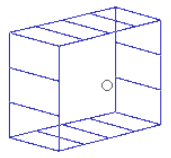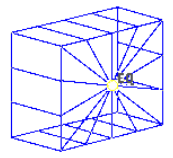# Equations Panel

Use the Equations panel to create, review, and update equations.

Location: Analysis page

Equations are displayed as lines between the dependent node and the independent node(s) with EQ displayed at the dependent node of the equation.

Equations are used in Nastran as MPC (Multipoint Constraint Equation) or in Abaqus as *equation.

Equations are organized into load collectors.

## Create Subpanel

The dependent node has one DOF and weight, whereas all the independent nodes have up to six DOFs and weights. Use the update subpanel to change the values of the individual nodes.Figure 1. Dependent Node SelectedFigure 2. Independent Node SelectedFigure 3. Equation Created
Option Action
dependent: node selector Select the node where the dependent end of the equation is to be created.
independent: nodes selector Select the nodes where the independent ends of the equation are to be created.
dependent node: dof1-6 Select DOF(s) to apply to the dependent node.
w Dependent node weight
dependent node: dof1-6 Select the DOF(s) to apply to the independent nodes.
w Specify an independent node weight.
constant Appears in the Abaqus solver interface only.

## Update Subpanel

From the Update subpanel you can edit the connectivity, DOFs, weight, and constants for each node of the equation.

Each update process must be completed before initiating another, that is you must click update after making changes to the connectivity before making changes to the DOFs or weight. The message "The changes to the graphic menu items have not been applied. Proceed is displayed if you perform editing in one category and attempt to start another before you click update.
Option Action
equation selector Select the equation to update.
connectivity Update the connectivity or add or remove nodes.
dofs and weights Update the connectivity or add or remove nodes
dependent node: dof1-6 Select the DOF(s) to update on the dependent node.
w Specify the dependent node weight.
independent node: dof1-6 Select the DOF(s) to apply to the independent nodes.
w Specify the independent node weight.
set all nodes / set single node
set all nodes
Apply the changes to the dependent DOF and weight and the independent DOFs and weights to the whole equation.
set single node
Apply the changes to individual nodes.
constant Appears in the Abaqus solver interface only.

## Command Buttons

Button Action
create Create the equation.
update Update the equation with the changes made.
reject Reject the most recently made changes.
card edit Open the Card Editor for the currently selected card image.
review Display information at each node, including whether it is the dependent or independent node, and the dofs and weight applied at the node.
return Exit the panel.